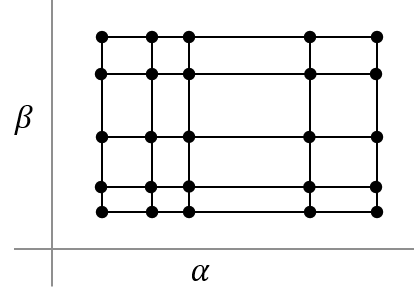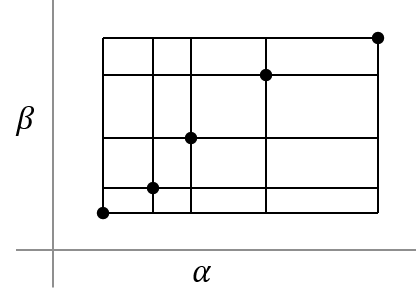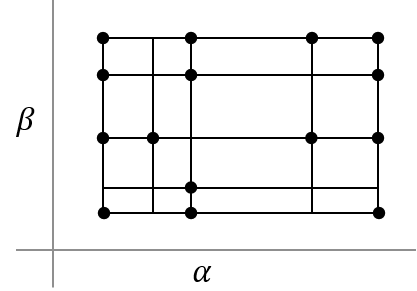## Linear Parameter-Varying Models

### What are Linear Parameter-Varying Models?

A linear parameter-varying (LPV) system is a linear state-space model whose dynamics vary as a function of certain time-varying parameters called scheduling parameters. In MATLAB®, an LPV model is represented in a state-space form using coefficients that are parameter dependent.

Mathematically, an LPV system is represented as:

 $\begin{array}{l}dx\left(t\right)=A\left(p\right)x\left(t\right)+B\left(p\right)u\left(t\right)\\ y\left(t\right)=C\left(p\right)x\left(t\right)+D\left(p\right)u\left(t\right)\\ x\left(0\right)={x}_{0}\end{array}$ (1)

where

• `u(t)` are the inputs

• `y(t)` the outputs

• `x(t)` are the model states with initial value `x0`

• $dx\left(t\right)$ is the state derivative vector $\stackrel{˙}{x}$ for continuous-time systems and the state update vector $x\left(t+\Delta T\right)$ for discrete-time systems. ΔT is the sample time.

• `A(p)`, `B(p)`, `C(p)` and `D(p)` are the state-space matrices parameterized by the scheduling parameter vector `p`.

• The parameters `p = p(t)` are measurable functions of the inputs and the states of the model. They can be a scalar quantity or a vector of several parameters. The set of scheduling parameters define the scheduling space over which the LPV model is defined.

#### Grid-Based LPV Model

A common way of representing LPV models is as an interpolated array of linear state-space models. A certain number of points in the scheduling space are selected, usually forming a regular grid. An LTI system is assigned to each point, representing the dynamics in the local vicinity of that point. The dynamics at scheduling locations in between the grid points is obtained by interpolation of LTI systems at neighboring points.

For example, the aerodynamic behavior of an aircraft is often scheduled over a grid of incidence angle (α) and wind speed (V) values. For each scheduling parameter, a range of values is chosen, such as α = 0:5:20 degrees, V = 700:100:1400 m/s. For each combination of (α,V) values, a linear approximation of the aircraft behavior is obtained. The local models are connected as shown in the following figure:Each donut represents a local LTI model, and the connecting curves represent the interpolation rules. The abscissa and ordinate of the surface are the scheduling parameters (α, V).

This form is sometimes called the grid-based LPV representation. This is the form used by the LPV System block. For meaningful interpolations of system matrices, all the local models must use the same state basis.

#### Affine Form of LPV Model

The LPV system representation can be extended to allow offsets in `dx`, `x`, `u` and `y` variables. This form is known as affine form of the LPV model. Mathematically, the following represents an LPV system:

 $\begin{array}{l}dx\left(t\right)=A\left(p\right)x\left(t\right)+B\left(p\right)u\left(t\right)+\left(\overline{dx}\left(p\right)-A\left(p\right)\overline{x}\left(p\right)-B\left(p\right)\overline{u}\left(p\right)\right)\\ y\left(t\right)=C\left(p\right)x\left(t\right)+D\left(p\right)u\left(t\right)+\left(\overline{y}\left(p\right)-C\left(p\right)\overline{x}\left(p\right)-D\left(p\right)\overline{u}\left(p\right)\right)\\ x\left(0\right)={x}_{0}\end{array}$ (2)

are the offsets in the values of `dx(t)`, `x(t)`, `u(t)` and `y(t)` at a given parameter value `p = p(t)`.

To obtain such representations of the linear system array, linearize a Simulink® model over a batch of operating points (see Batch Linearization (Simulink Control Design).) The offsets correspond to the operating points at which you linearized the model.

You can obtain the offsets by returning additional linearization information when calling functions such as `linearize` (Simulink Control Design) or `getIOTransfer` (Simulink Control Design). You can then extract the offsets using `getOffsetsForLPV` (Simulink Control Design). For an example, see LPV Approximation of Boost Converter Model (Simulink Control Design).

In the affine representation, the linear model at a given point ```p = p*``` in the scheduling space is:

`$\begin{array}{l}d\Delta x\left(t,{p}^{*}\right)=A\left({p}^{*}\right)\Delta x\left(t,{p}^{*}\right)+B\left({p}^{*}\right)\Delta u\left(t,{p}^{*}\right)\\ \Delta y\left(t,{p}^{*}\right)=C\left({p}^{*}\right)\Delta x\left(t,{p}^{*}\right)+D\left({p}^{*}\right)\Delta u\left(t,{p}^{*}\right)\end{array}$`

The states of this linear model are related to the states of the overall LPV model (Equation 2) by $\Delta x\left(t,{p}^{*}\right)=x\left(t\right)-\overline{x}\left({p}^{*}\right)$. Similarly, $\Delta y\left(t,{p}^{*}\right)=y\left(t\right)-\overline{y}\left({p}^{*}\right)$ and $\Delta u\left(t,{p}^{*}\right)=u\left(t\right)-\overline{u}\left({p}^{*}\right)$.

### Regular vs. Irregular Grids

Consider a system that uses two scheduling parameters, α and β. When α and β vary monotonically, a regular grid is formed, as shown in the next figure. The state space array contains a value at every combination of α and β values. Regular grid does not imply uniform spacing between values.When parameters co-vary, that is, α and β increase together, an irregular grid is formed. The system array parameters are available only along the diagonal in the parameter plane.If certain samples are missing from an otherwise regular grid, the grid is considered to be irregular.### Use Model Arrays to Create Linear Parameter-Varying Models

The array of state-consistent linear models that define an LPV model are represented by an array of state-space model objects. For more information on model arrays, see Model Arrays.

The system array size is equal to the grid size in scheduling space. In the aircraft example, α takes 5 values in the 0–20 degrees range and V takes 8 values in the 700–1400 m/s range. If you define a linear model at every combination of (α,V) values (i.e., the grid is regular), the grid size is 5-by-8. Therefore, the model array size must be 5-by-8.

The information about scheduling parameters is attached to the linear model array using its `SamplingGrid` property. The value of the `SamplingGrid` property must be a structure with as many fields as there are scheduling parameters. For each field, the value must be set to all the values assumed by the corresponding variable in the scheduling space.

For the aircraft example, you can define the `SamplingGrid` property as:

```Alpha = 0:5:20; V = 700:100:1400; [Alpha_Grid,V_Grid] = ndgrid(Alpha, V); linsysArray.SamplingGrid = struct('Alpha',Alpha_Grid,'V',V_Grid); ```

### Approximate Nonlinear Systems using LPV Models

In the same way as a linear model provides the approximation of system behavior at a given operating condition, an LPV model provides the approximation of the behavior over a span on operating conditions. A common approach for constructing the LPV model is by batch trimming and linearization, followed by stacking the local models in a state-space model array.

Note

When obtaining linear models by linearization, do not reduce or alter the state variables used by the models.

The operating region is usually of a high dimension because it consists of all the input and state variables. Generating or interpolating local models in such high-dimensional spaces is usually infeasible. A simpler approach is to use a small set of scheduling parameters as a proxy for the operating space variables. The scheduling parameters are derived from the inputs and state variables of the original system. You must choose the values carefully so that for a fixed value of the scheduling parameters, the system behavior is approximately linear. This approach is not always possible.

Consider a nonlinear system described by the following equations:

Suppose you use as a scheduling variable. At a given time instant t = t0, you have:

Thus, the dynamics are linear (affine) in the neighborhood of a given value of $\stackrel{˙}{x}$. The approximation holds for all time spans and values of input u as long as of $\stackrel{˙}{x}$ does not deviate much from its nominal value at sampling point t0. Note that scheduling on input u or states x1 or x2 does not help locally linearize the system. Therefore, they are not good candidates for scheduling parameters.

For an example of this approach, see Approximate Nonlinear Behavior Using Array of LTI Systems (Simulink Control Design).

### Applications of Linear Parameter-Varying Models

#### Modeling Multimode Dynamics

You can use LPV models to represent systems that exhibit multiple modes (regimes) of operation. Examples of such systems include colliding bodies, systems controlled by operator switches, and approximations of systems affected by dry friction and hysteresis effects. For an example, see Using LTI Arrays for Simulating Multi-Mode Dynamics.

#### Proxy Modeling for Faster Simulations

This approach is useful for generating surrogate models that you can use in place of the original system for enabling faster simulations, reducing memory footprint of target hardware code, and hardware-in-loop (HIL) simulations. You can also use surrogate models of this type for designing gain-scheduled controllers and for initializing the parameter estimation tasks in Simulink. For an example of approximating a general nonlinear system behavior by an LPV model, see Approximate Nonlinear Behavior Using Array of LTI Systems (Simulink Control Design).

LPV models can help speed up the simulation of physical component based systems, such as those built using Simscape™ Multibody™ and Simscape Electrical™ Power Systems software. For an example of this approach, see LPV Approximation of Boost Converter Model (Simulink Control Design).